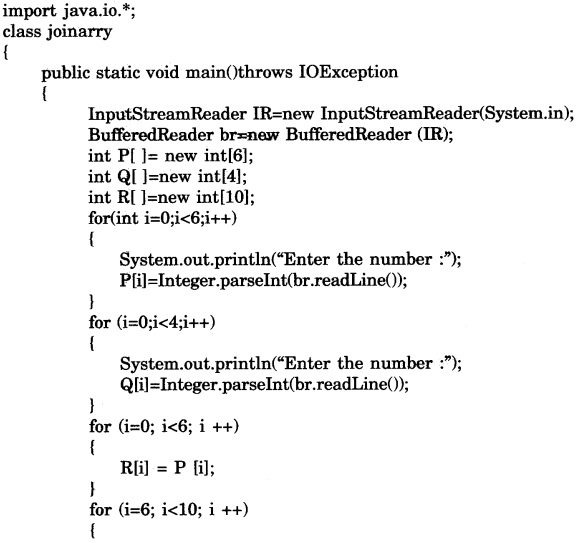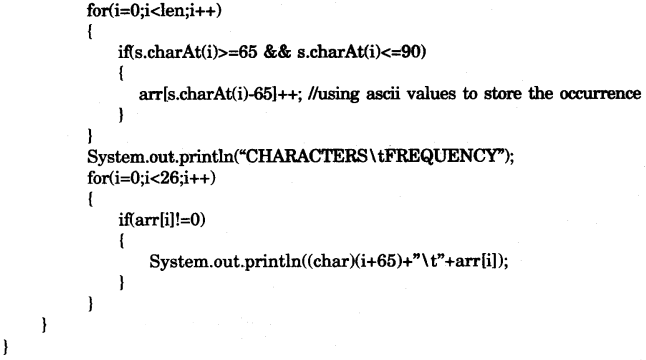## ICSE Computer Applications Previous Year Question Paper 2010 Solved for Class 10

ICSE Paper 2010
COMPUTER APPLICATIONS

(Two Hours)
Answers to this Paper must be written on the paper provided separately.
You will not be allowed to write during the first 15 minutes.
This time is to be spent in reading the question paper.
The time given at the head of this Paper is the time allowed for writing the answers.
This paper is divided into two Sections.
Attempt all questions from Section A and any four questions from Section B.
The intended marks for questions or parts of questions are given in brackets [ ].

Section ‘A’ (40 Marks)

(Attempt all questions)

Question 1:
(a) Define the term Byte code. 
(b) What do you mean by type conversion ? How is implicit conversion different from explicit conversion ? 
(c) Name two jump statements and their use. 
(d) What is an Exception ? Name two Exception handling blocks. 
(e) Write two advantages of using functions in a program. 

(a) Byte code is a term which has been used to denote various forms of instruction sets designed for efficient execution by a software interpreter as well as being suitable for further compilation into machine code.

(b) In Java, type conversions are performed automatically when the type of the expression on the right hand side of an assignment operation can be safely promoted to the type of the variable on the left hand side of the assignment. Thus we can safely assign :
byte to short to int to long to float to double
for example
long myLongInteger; // 64 bit long integer
int, myInteger; // 32 bit standard integer
myLongInteger = myInteger;
The extra storage associated with the long integer, in the above example, will simply be padded with extra zeros.
Explicit Conversion (Casting)
The above will not work the other way round. For example we cannot automatically convert a long to an int because the first requires more storage than the second and consequently information may be lost. To force such a conversion we must carry out an explicit conversion (assuming of course that the long integer will fit into a standard integer). This is done using a process known as a type cast :
For example :
myInteger = (int) myLongInteger;
This tells the compiler that the type of myLongInteger must be temporarily changed to an int when the given assignment statement is processed. Thus, the cast only lasts for the duration of the assignment.

(c) The two jump statements are break and continue. Their use is defined as follows :
Some times we need to exit from a loop before the completion of the loop then we use break statement and exit from the loop and loop is terminated. Sometimes we need to iterate the loop before it finishes all the statements inside a loop then we use continue statement and jump to the next iteration of a loop.

(d) An exception is an event that .occurs during the execution of a program that disrupts the normal flow of instructions.
The two exception handling blocks are try and catch.
(i) The try block identifies a block of code in which an exception can occur.
(ii) The catch block identifies a block of code, known as an exception handler that can handle a particular type of exception.

(e) Two advantages of a function in a program:
(i) Debugging is easier.
(ii) It is easier to understand the logic involved in the program.

Question 2:
(a) State the purpose and return data type of the following String functions :
(i) indexOf().
(ii) compareTo(). 
(b) What is the result stored in x, after evaluating the following expression :
int x = 5; x=x++*2+3* – -x; 
(c) Differentiate between static and non-static data members. 
(d) Write the difference between length and length() functions. 
(e) Differentiate between private and protected visibility modifiers. 

(a) (i) This method returns the first occurance of a character or a sub-string in the string. It returns integer value.
e.g. : int r = s1.indexOF(“DAY”);
string s1 = “HAPPY BIRTHDAY”;
System.out.println(r);
Output : 11
(ii) This method compare the invoking string with string object str exicographically & returns an integer value.
e.g. : string s1 = “GOD”;
if (s1.compare To (“OQ”)= = 0)
System.out.println (“equal”);
Output : <Nothing>
The result of the comparison is returned and is interpreted as shown here :

 Value returned Meaning Less than zero The invoking string is less than str. Greater than zero The invoking string is greater than str. Zero The two string are equal.

(b) The value of x is :
x=x++*2 + 3 * – -x
//sifter putting the values using precedence order x=5 *2 + 3*5
x =10 + 15;
//finally
x=25.

(c) A static variable is associated with the class sis a whole rather than with specific instances of a class.
Non-static, variables take different values with each object/instance.
(d) Both returns an integer value. length property is used with an array to get the number of elements of array wheres length() method is used with String to get the length of the stored string.
(e) The data members defined under Private visibility modifiers are visible only to the class to which they belong. Where as the data members defined under Protected visibility modifiers are visible not only to the class to which they belong but also to any subclasses using inheritance.

Question 3:
(a) What do you understand by the term data abstraction ? Explain with an example. 

(b) What will be the output of the following code ?
(i) int m=2;
int n=15;
for(int i = 1; i<5; i++);
m++; – -n;
System.out.println(“m=”+m);
System.out.println(“n-”+n); 
(ii) char x=‘A’; int m;
m= (x==’a’) ? ‘A’: ‘a’;
System.out.println(“m=” +m); 

(c) Analyse the following program segment and determine how many times the loop will be executed and what will be the output of the program segment.
int k=1 ,i=2;
while(++i<6)
k*=i;
System.outprintln(k); 

(d) Give the prototype of a function check which receives a character ch and an integer n and returns true or false. 

(e) State two features of a constructor. 

(f) Write a statement each to perform the following task on a string :
(i) Extract the second last character of a word stored in the variable wd. 
(ii) Check if the second character of a string str is in uppercase. 

(g) What will the following functions return when executed ?
(i) Math.max(-17, -19)
(ii) Math.Ceil(7.8) 

(h) (i) Why is an object called an instance of a class ?
(ii) What is the use of the keyword import ? 

(a) Data Abstraction refers to die act of representing the essential features without including the background details complexity.

(b) (i) After executing this code the output will be:
m=3
n=14
(ii) After executing this code the output will be: m=97

(c) After analyzing the code it was observed that the loop executed 3 times and the output is 60.

(d) boolean check (char ch, int n)

(e) Two features of a constructor:
1. Its names is same as the name of the class.
2. It is automatically executed at the time of object creation.

(f) (i) char ch=wd.charAt(wd.length()-2);
(ii) if(Character.isUpperCase(str.charAt( 1)))
{
//statement;
}

(g) (i) It will return -17.
(ii) It will return 8.0

(h) (i) A class is the structure of an object, meaning the definition of all items that an object is made up of. An object is therefore the “example” of a class. In reality, an object is an instance of a class, which is why can use interchange the terms object or instance.
(ii) import keyword is used to import the source code already designed and kept under some package in the form of a class. It helps us to use the user defined code or predefined code within other programs without creating the code again.

SECTION B (60 Marks)

Attempt any four questions from this Section.
The answers in this Section should consist of the Programs in either Blue environment or any program environment with Java as the base.
Each program should be written using Variable descriptions! Mnemonic Codes such that the logic of the program is clearly depicted.
Flow-Charts and Algorithms are not required.

Question 4:
Write a program to perform binary search on a list of integers given below, to search for an element input by the user, if it is found display the element along with its position, otherwise display the message “Search element not found”.
5, 7, 9, 11, 15, 20, 30, 45, 89, 97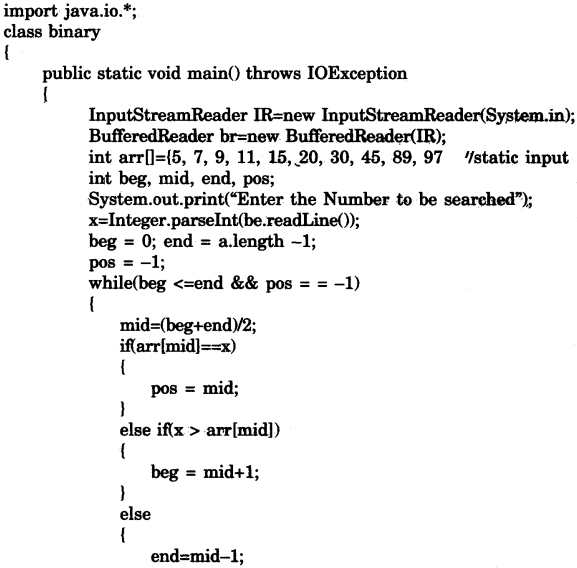Question 5:
Define a class student described as below:
Data members I instance variables:
name, age, m1, m2, m3 (marks in 3 subjects), maximum, average Member methods
(i) A parameterized constructor to initialize the data members
(ii) To accept the details of a student
(iii) To compute the average and the maximum out of three marks
(iv) To display the name, age, marks in three subjects, maximum and average.
Write a main method to create an object of a class and call the above member methods.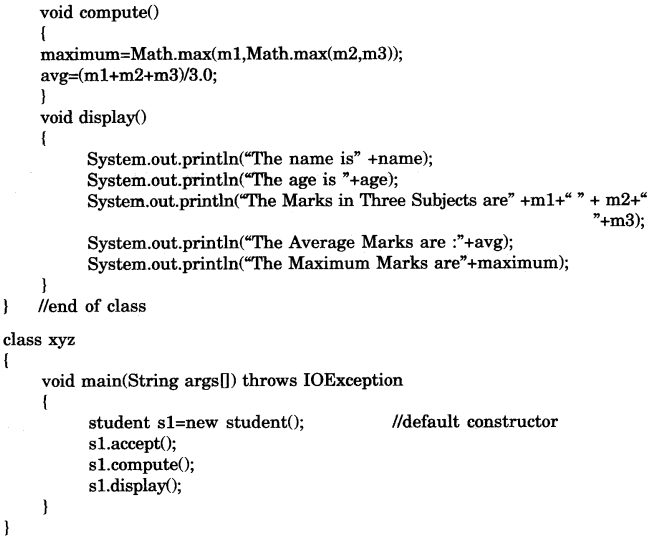Question 6:
Shasha Travels Pvt. Ltd. gives the following discount to its customers:

 Ticket amount Discount Above Rs. 70000 18% Rs. 55001 to Rs. 70000 16% Rs. 35001 to Rs. 55000 12% Rs. 25001 to Rs. 35000 10% less than Rs. 25001 2%

Write a program to input the name and ticket amount for the customer and calculate the discount amount and net amount to he paid. Display the output in the following format for each customer: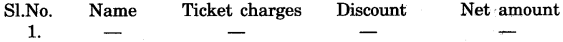(Assume that there are 15 customers, first customer is given the serial no (SI. No.) 1, next customer 2 …….. and so on)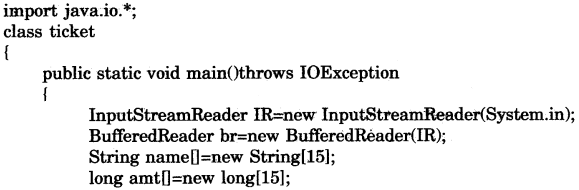Question 7:
Write a menu driven program to accept a number and check and display whether it is a prime number or not OR an automorphic number or not. (Use switch- case statement)
(a) Prime number: a number is said to be a prime number if it is divisible only by 1 and itself and not by any other number.
Example : 3, 5, 7, 11, 13 etc.,
(b) Automorphic number: An automorphic number is the number which is contained in the last digit(s) of its square.
Example 25 is an automorphic number as its square is 625 and 25 is present as the last two digits.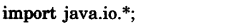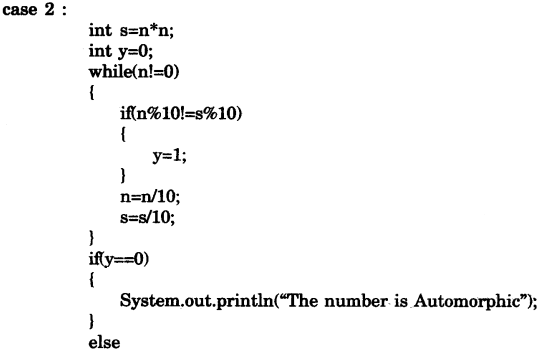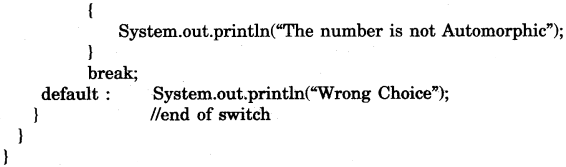Question 8:
Write a program to store 6 elements in any array P, and 4 elements in an array Q and produce a third array R, containing all the elements of array P and Q. Display the resultant array.